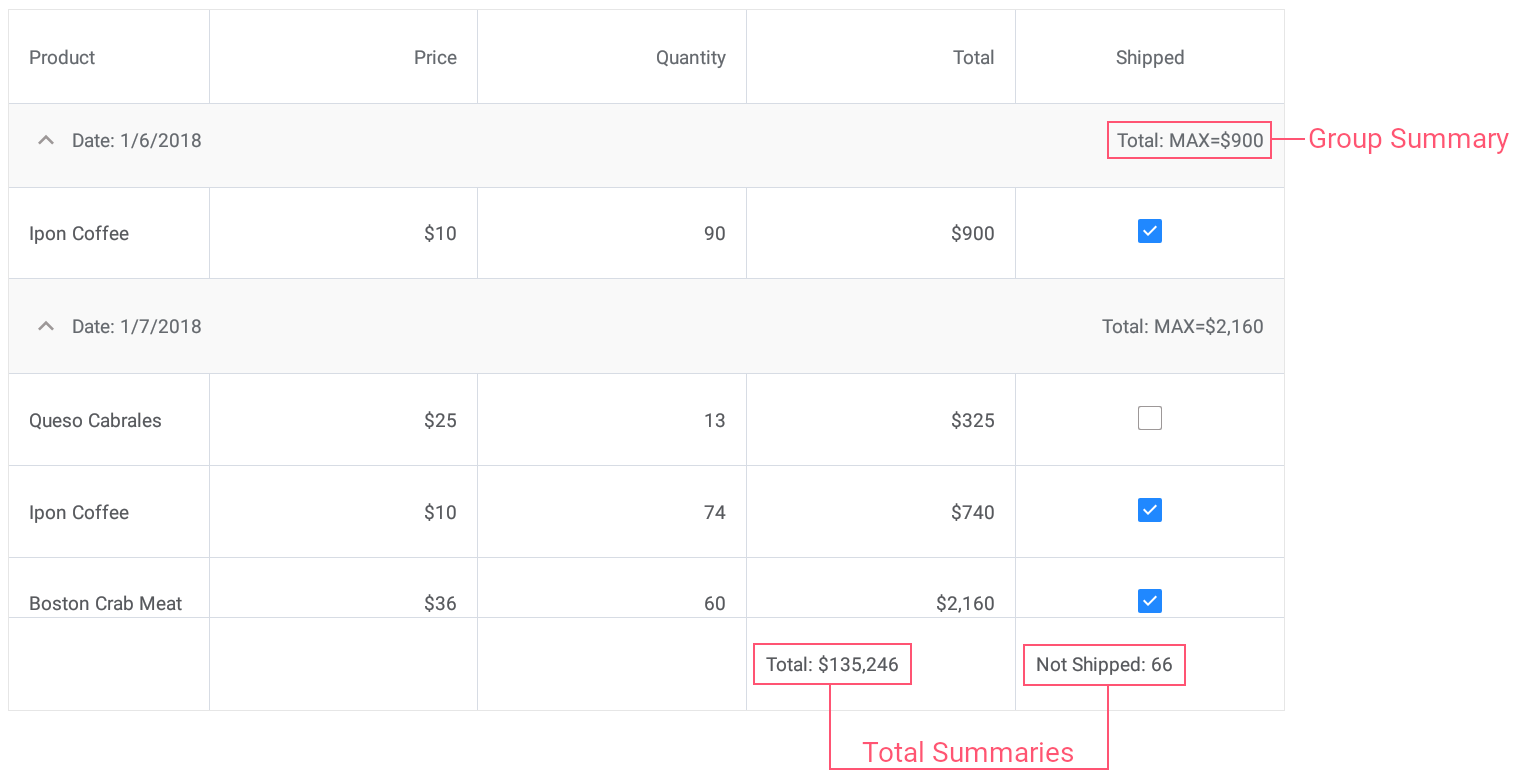21.1 (EAP/Beta)
20.2
20.1
19.2
19.1
1.1
The page you are viewing does not exist in version 1.1. This link will take you to the root page.

# GridColumnSummary.Type Property

Gets or sets the aggregate function type.

Namespace: DevExpress.XamarinForms.DataGrid

Assembly: DevExpress.XamarinForms.Grid.dll

## Declaration

``````[XtraSerializableProperty]
public SummaryType Type { get; set; }``````

## Property Value

Type Description
SummaryType

A SummaryType enumeration value that specifies the aggregate function type.

Available values:

Name Description
None

Disables summary value calculation.

Sum

The sum of all values in a column.

Min

The minimum value in a column.

Max

The maximum value in a column.

Count

The record count.

Average

The average value of a column.

Custom

Specifies whether data summaries should be calculated according to a custom rule specified in the DataGridView.CalculateCustomSummary event handler.

## Remarks

Use the Type property to specify the aggregate function that should be used to calculate the summary value. There are five predefined aggregate functions for grid data summaries: Sum, Min, Max, Average and Count.

To implement and apply a custom aggregate function, set the Type property to Custom and handle the DataGridView.CalculateCustomSummary event.

## Examples

This example demonstrates how to use predefined aggregate functions and custom rule to calculate group and total summaries for grid columns.• Set the group summary to display the maximum Total value for each group of records.
• Set the total summary to calculate the sum of values in the Total column.
• Set the custom total summary to count the number of orders whose value in the Shipped column is false (to count orders that are not shipped).
``````<dxg:DataGridView x:Name="grid" ItemsSource="{Binding Orders}"
CalculateCustomSummary="OnCalculateCustomSummary">
<!-- ... -->
<dxg:DataGridView.GroupSummaries>
<dxg:GridColumnSummary FieldName="Total" Type="Max"/>
</dxg:DataGridView.GroupSummaries>

<dxg:DataGridView.TotalSummaries>
<dxg:GridColumnSummary FieldName="Total" Type="Sum"
DisplayFormat="Total: {0:C0}"/>
<dxg:GridColumnSummary FieldName="Shipped" Type="Custom"
DisplayFormat="Not Shipped: {0}"/>
</dxg:DataGridView.TotalSummaries>
</dxg:DataGridView>
``````
``````int count;
// ...

void OnCalculateCustomSummary(object sender, CustomSummaryEventArgs e) {
if (e.FieldName.ToString () == "Shipped")
if (e.IsTotalSummary){
if (e.SummaryProcess == CustomSummaryProcess.Start) {
count = 0;
}
if (e.SummaryProcess == CustomSummaryProcess.Calculate) {
if (!(bool)e.FieldValue)
count++;
e.TotalValue = count;
}
}
}
``````